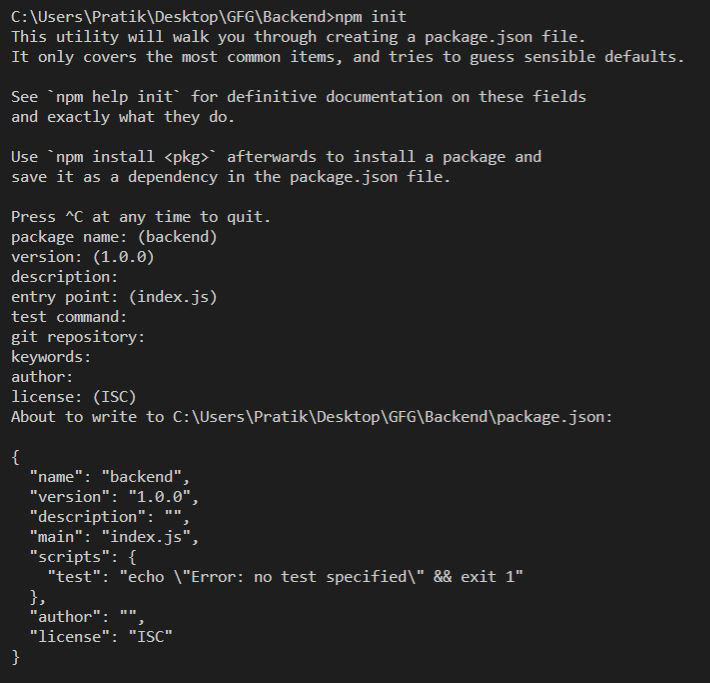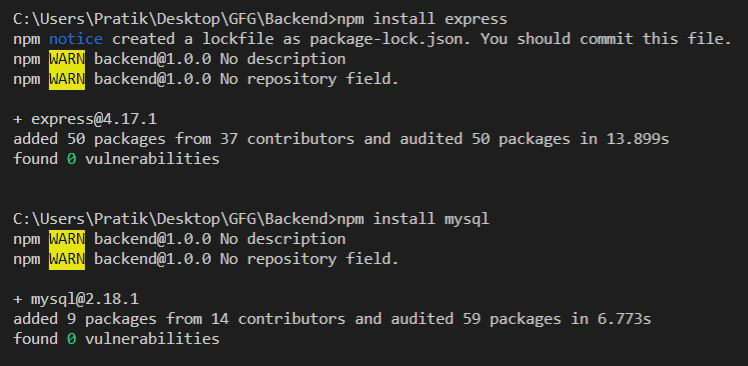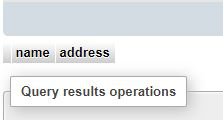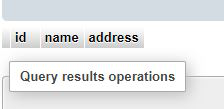Related Articles
NodeJS MySQL Create Table
• Difficulty Level : Medium
• Last Updated : 08 Feb, 2021

Introduction: Learn to create a table in MySQL database using NodeJS. We will see how to use the Create Table command in NodeJS using the MySQL module.

Prerequisite: Introduction to NodeJS MySQL

Setting up environment and Execution:

Step 1: Create a NodeJS Project and initialize it using the following command:

`npm init`Step 2: Install the express and mysql modules using the following command:

```npm install express
npm install mysql```File Structure: Our file structure will look like the following:Example: Create and export MySQL Connection Object as shown below:

Filename: sqlConnection.js

## Javascript

 `const mysql = require(``"mysql"``);`` ` `let db_con  = mysql.createConnection({``    ``host: ``"localhost"``,``    ``user: ``"root"``,``    ``password: ``''``,``    ``database: ``'gfg_db'``});`` ` `db_con.connect((err) => {``    ``if` `(err) {``      ``console.log(``"Database Connection Failed !!!"``, err);``    ``} ``else` `{``      ``console.log(``"connected to Database"``);``    ``}``});`` ` `module.exports = db_con;`

Example 1: Creating a table without a primary key.

## index.js

 `const express = require(``"express"``);``const database = require(``'./sqlConnection'``);`` ` `const app = express();`` ` `app.get(``"/"``, (req, res) => {``   ` `  ``let tableName = ``'gfg_table'``;`` ` `  ``// Query to create table``  ``let query = `CREATE TABLE \${tableName} (``    ``name VARCHAR(255), address VARCHAR(255))`;`` ` `  ``database.query(query, (err, rows) => {``      ``if``(err) ``return` `res.status(500)``          ``.send(``"Table Creation Failed"``);`` ` `      ``return` `res.send(```Successfully Created Table - \${tableName}`);``  ``})``});`` ` `app.listen(5000, () => {``  ``console.log(`Server is up and running on 5000 ...`);``});`

Run the index.js file using the following command:

`node index.js`

Output:

`Successfully Created Table - gfg_table`Example 2: Create a Table with an auto-increment primary key.

## index.js

 `const express = require(``"express"``);``const database = require(``'./sqlConnection'``);`` ` `const app = express();`` ` `app.get(``"/"``, (req, res) => {``     ` `    ``let tableName = ``'gfg_table'``;`` ` `    ``// Query to create table``    ``let query = `CREATE TABLE \${tableName} ``        ``(id INT AUTO_INCREMENT PRIMARY KEY, ``        ``name VARCHAR(255), address VARCHAR(255))`;`` ` `    ``database.query(query, (err, rows) => {``        ``if``(err) ``return` `res.status(500)``            ``.send(``"Table Creation Failed"``);`` ` `        ``return` `res.send(```Successfully Created Table - \${tableName}`);``    ``})``});`` ` `app.listen(5000, () => {``  ``console.log(`Server is up and running on 5000 ...`);``});`

Run the index.js file using the following command:

`node index.js`

Output:

`Successfully Created Table - gfg_table`Attention reader! Don’t stop learning now. Get hold of all the important DSA concepts with the DSA Self Paced Course at a student-friendly price and become industry ready.

My Personal Notes arrow_drop_up